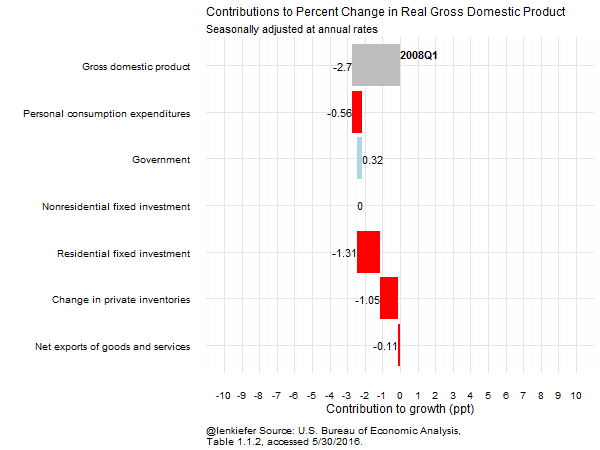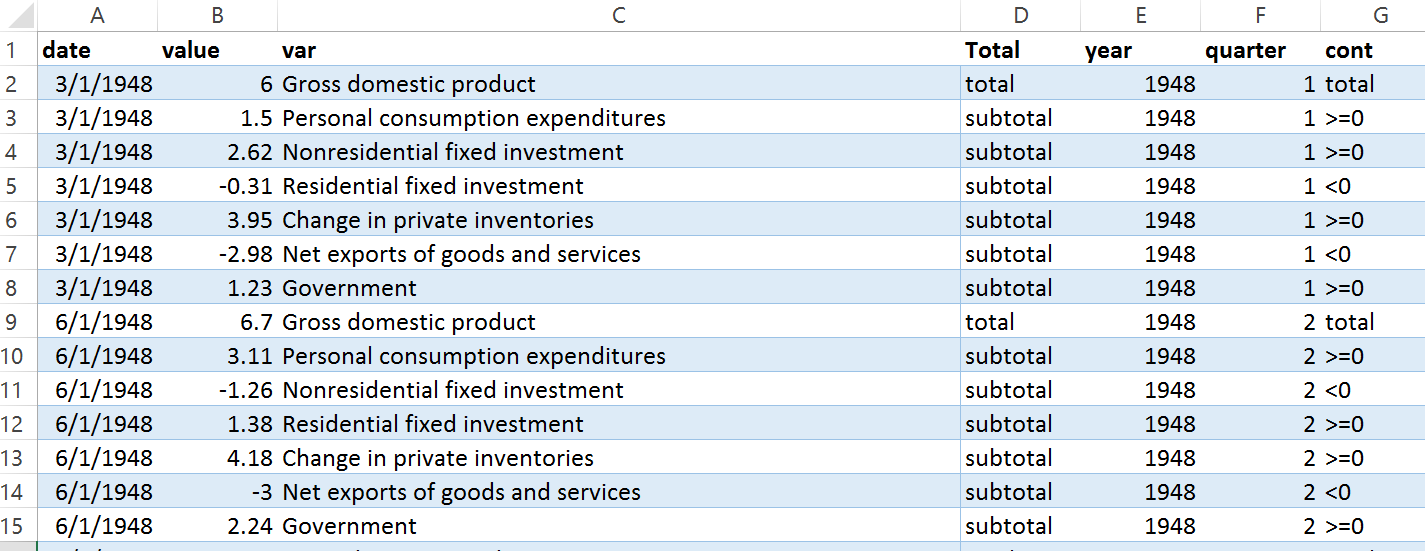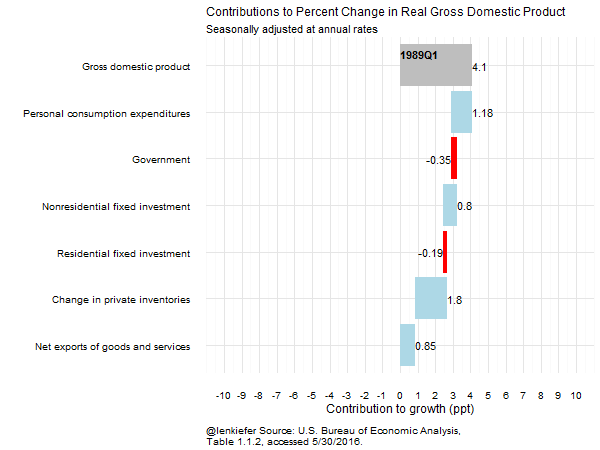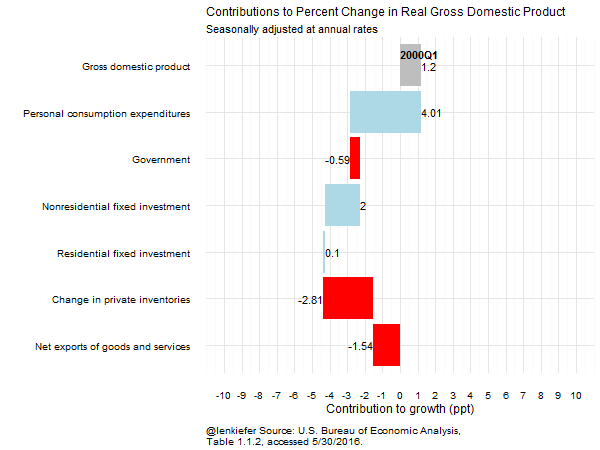# More tweenr animation examples

IN THIS POST I WANT TO PROVIDE some additional examples of using tweenr and gganimate to create nice smooth transitions in an animated GIF. In this post we’ll look at an animated waterfall chart.

For this data I’m going to use the National Income and Products Accounts from the U.S. Bureau of Economic Analysis (BEA). Specifically we’ll be looking at the contributions to growth in Real Gross Domstic Product, which you can find here.

Ultimately, we will end up with this:## How to construct the chart

I found a nice example of how to make a waterfall chart in R that I used. The basic idea is to draw a rectangle shifted over to the right (or left) depending on the data. The basic idea is to show how individual contributions contribute positivley or negatively to a whole. In this case, we’re going to look at how various components of the economy affect total economic growth in a given quarter.

### Setup

If you follow the link above, you can download a spreadsheet from BEA. I had to do a bit of wrangling, which I happened to do in Excel. I’m not writing up the data wrangling bits here, but you can check out my earlier post on house prices to get some ideas.

After some wrangling, I ended up with data that looked like this:Next I imported these data into R as a text file name gdpc.txt. We’ll pick up the R code from there:

{% highlight r gdata <- fread(“gdpc.txt”) gdata$date<-as.Date(gdata$date, format=“%m/%d/%Y”) gdata[, avgc:=mean(value), by=var] #I’ll want to order some factors, so i’m computed average contribution gdata<-gdata[order(date,avgc),]

# I’m taking advantage of data.table to compute the data

gdata[Total !=“total”, end:=cumsum(value), by=date] gdata[Total==“total”, end:=0, by=date] gdata[,start:=shift(end,1,fill=0), by=date] gdata[,id:=1:.N,,by=date]

# I include the variable mjust to control where the label shows up in the chart

gdata[, myjust:=ifelse(value<0,1,0)]

# I’m creating a label, which for the total column needs to start at “start”, not “end”

gdata[, lp:=ifelse(Total==“total”,start,end)]

# A date label for the total bar

gdata[,dlabel:=factor(paste(year,“Q”,quarter,sep=“”))]

# I need to convert the character variables to factors to use tweenr

gdata[,cont:=factor(cont)] # cont is used to color the bars

# If the data were different we’d have to choose a different path to reoder the factors

gdata$var<-factor(gdata$var, levels=gdata$var[order(gdata$avgc)]) {% endhighlight

These data are now ready to draw the waterfall chart. Let’s make one:

# create a function to make a list of data frames

myf<-function(d){as.data.frame(gdata[date==d, list(date,end,start,id,myjust,lp,var,cont,value,dlabel,year,avgc)])}

# use lapply to generate the list of data sets:

my.list<-lapply(myd,myf)

# apply tweenr

tf <- tween_states(my.list, tweenlength= 2, statelength=1, ease=rep(‘cubic-in-out’,64),nframes=250)

# convert variable lables to factor, and order by average contribution so they go from big to small

tf$var<-factor(tf$var, levels=tf$var[order(tf$avgc)]) #conver to data table tf<-data.table(tf)

# Make animation using gganimate:

p<- ggplot(data=tf, aes(x=var, y=lp,fill = cont,label=value,frame=.frame)) + geom_rect(aes(x = var, xmin = id - 0.45, xmax = id + 0.45, ymin = end,ymax = start)) + theme_minimal()+ coord_flip()+ #flip bars to go horizontal to allow more room for labels geom_text(data=tf[date %in% myd , ] ,hjust=tf[date %in% myd , ]\$myjust)+ labs(title=“Contributions to Percent Change in Real Gross Domestic Product”,x=“”,y=“”, subtitle=“Seasonally adjusted at annual rates”, caption=“@lenkiefer Source: U.S. Bureau of Economic Analysis, Table 1.1.2, accessed 5/30/2016.“)+ scale_fill_manual(name=“”,values=c(“red”,“lightblue”,“gray”))+ theme(plot.title=element_text(size=12))+ geom_text(data=tf[cont==“total” , ] ,aes(y=0,x=7.25,label=dlabel),hjust=0,size=4,fontface=“bold”) + theme(plot.caption=element_text(hjust=0,vjust=1,margin=margin(t=10),size=10))+ theme(legend.justification=c(0,0), legend.position=“none”)

# Now animate!

gg_animate(p, “gif1.gif”, title_frame = F, ani.width = 600, ani.height = 450, interval=.01) {% endhighlight

You can adjust the dates to look at different periods:

### Around the early 1990s recession### Around the early 2000s recession